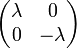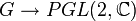# Projective representation theory of Klein four-group

(diff) ← Older revision | Latest revision (diff) | Newer revision → (diff)
This article gives specific information, namely, projective representation theory, about a particular group, namely: Klein four-group.
View projective representation theory of particular groups | View other specific information about Klein four-group

This article describes the projective representation theory of the Klein four-group in characteristic zero, which we call$G$.

First, note that the Schur multiplier$H^2(G,\mathbb{C}^\ast)$ is isomorphic to cyclic group:Z2. For more, see group cohomology of Klein four-group.

## Summary description in terms of linear representations of a Schur covering group

We can take dihedral group:D8 as a Schur covering group for the Klein four-group (we could also alternatively take the quaternion group). Thus, all projective representations of the Klein four-group arise from ordinary representations of dihedral group:D8. Below is a complete description of the list of irreducible projective representations and the corresponding ordinary representations of dihedral group:D8.

Representation Degree Corresponding element of Schur multiplier (i.e., corresponding cohomology class) Number of linear representations of dihedral group:D8 that descend to this (see linear representation theory of dihedral group:D8) List of these linear representations
trivial 1 trivial 4 all the one-dimensional representations
nontrivial 2 nontrivial 1 faithful irreducible representation of dihedral group:D8

## Projective representations for trivial cohomology class

For the trivial cohomology class, there is a unique irreducible projective representation, namely the one-dimensional projective representation induced by the trivial representation. All one-dimensional representations are projectively equivalent to the trivial representation, and they all induce this projective representation.

In addition to the four irreducible representations of the Klein four-group, any one-dimensional representation of any group admitting the Klein four-group as a quotient also induces the trivial projective representation on the Klein four-group.

## Projective representations for nontrivial cohomology class

There is a unique irreducible projective representation up to equivalence. This has degree two, and is described below. We denote by$e$,$a$,$b$,$c$ the four non-identity elements. Note that the values$\lambda$ used for each of the elements may differ from one another.

Element Arbitrary representative for projective equivalence class Generic element of projective equivalence class Nature of characteristic polynomial Nature of minimal polynomial Is the trace zero or nonzero?$e$$\begin{pmatrix} 1 & 0 \\ 0 & 1 \\\end{pmatrix}$$\begin{pmatrix} \lambda & 0 \\ 0 & \lambda \\\end{pmatrix}$$(t - \lambda)^2$$t - \lambda$ nonzero$a$$\begin{pmatrix} 0 & -1 \\ 1 & 0 \\\end{pmatrix}$$\begin{pmatrix} 0 & -\lambda \\ \lambda & 0 \\\end{pmatrix}$$t^2 - \lambda^2$$t^2 - \lambda^2$ zero$b$$\begin{pmatrix} 1 & 0 \\ 0 & -1 \\\end{pmatrix}$$\begin{pmatrix} \lambda & 0 \\ 0 & -\lambda \\\end{pmatrix}$$t^2 - \lambda^2$$t^2 - \lambda^2$ zero$c$$\begin{pmatrix} 0 & 1 \\ 1 & 0 \\\end{pmatrix}$$\begin{pmatrix} 0 & \lambda \\ \lambda & 0 \\\end{pmatrix}$$t^2 - \lambda^2$$t^2 - \lambda^2$ zero

This projective representation can be realized by choosing a two-dimensional faithful irreducible linear representations of a group of the form$K$ with a central subgroup$N$ such that$K/N \cong G$. To see how, note that by a corollary of Schur's lemma, all elements of$N$ must map to scalar matrices. so the map$K \to GL(2,\mathbb{C})$ induces a map$K/N \to PGL(2,\mathbb{C})$ and hence a map$G \to PGL(2,\mathbb{C})$. Here are some examples of choices of$K$ and$N$ (it also turns out that for the two-dimensional faithful irreducible representation to exist,$N$ must be precisely the center, because if the center were any bigger, then the whole group would be abelian and hence all irreducible representations would be one-dimensional):

Group$K$ Central subgroup$N$ as subgroup Isomorphism class of$N$ Cohomology group$H^2(G,N)$ for trivial group action Faithful irreducible two-dimensional representation of$G$ that works
dihedral group:D8 center of dihedral group:D8 cyclic group:Z2 second cohomology group for trivial group action of V4 on Z2 faithful irreducible representation of dihedral group:D8
quaternion group center of quaternion group cyclic group:Z2 second cohomology group for trivial group action of V4 on Z2 faithful irreducible representation of quaternion group
M16 center of M16 cyclic group:Z4 second cohomology group for trivial group action of V4 on Z4 faithful irreducible representation of M16
central product of D8 and Z4 center of central product of D8 and Z4 cyclic group:Z4 second cohomology group for trivial group action of V4 on Z4 faithful irreducible representation of central product of D8 and Z4